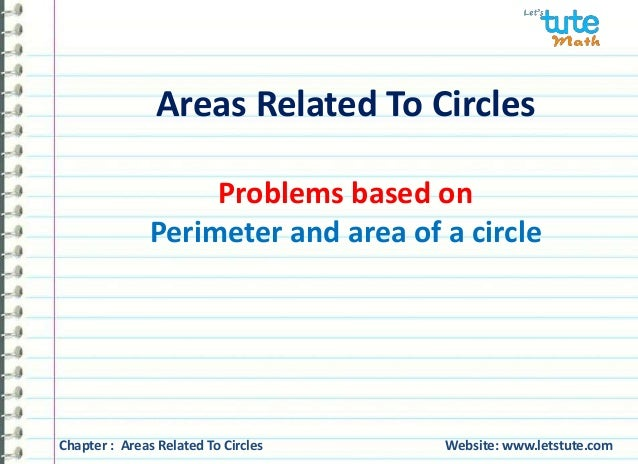Successfully reported this slideshow.Upcoming SlideShare
×

# Areas related to circles - Perimeter and area of a circle for class 10 maths.

6,923 views

Published on

Areas related to circles - Perimeter and area of a circle for class 10 maths.
Let's tute is an E-school or E- platform which is free for the student.Students will watch "MATHS" Videos for conceptual understanding.

Published in: Education
• Full Name
Comment goes here.

Are you sure you want to Yes No### Areas related to circles - Perimeter and area of a circle for class 10 maths.

1. 1. Areas Related To Circles Problems based on Perimeter and area of a circle Chapter : Areas Related To Circles Website: www.letstute.com
2. 2. Problems based on Perimeter and area of a circle Q) A wheel rotates 25000 times to cover a distance of 90 km. Find its radius. Given: Rotation of the wheel = 25000 times Distance covered = 90 km by the wheel To find: Radius of the wheel = ? ? Chapter : Areas Related To Circles Website: www.letstute.com
3. 3. Problems based on Perimeter and area of a circle Solution: Let ‘r’ be the radius of the wheel. ? Circumference of the wheel = Distance covered in one rotation. = 90 Km 2πr ∵ ퟗퟎ 퐊퐦= 90 x 1000 x 100 cm = 9000000 25000 = 360 cm 2πr = 360 cm Chapter : Areas Related To Circles Website: www.letstute.com
4. 4. Problems based on Perimeter and area of a circle 2 x 22 x r = 360cm r = 360x7 cm 2x22 = 180 x 7 22 = 90 x 7 11 = 630 11 r = 57.27 cm 7 Hence, the radius of the wheel is 57.27cm ? Chapter : Areas Related To Circles Website: www.letstute.com
5. 5. Problems based on Perimeter and area of a circle Q) The diameter of a cart wheel is 21 cm. How many revolutions will it make in moving 1.32 km? 21 cm Given: Diameter of the cart wheel = 21 cm To Find: Number of revolutions = ? made in 1.32 Km Chapter : Areas Related To Circles Website: www.letstute.com
6. 6. Problems based on Perimeter and area of a circle Solution: Let the radius of the cart wheel be ’r’. Thus, r = Diameter = 21 cm 2 2 Circumference of the cart wheel = 2πr = 2x22x21cm 7 2 = 462 7 = 66 cm 21 cm Chapter : Areas Related To Circles Website: www.letstute.com
7. 7. Problems based on Perimeter and area of a circle 21 cm Converting 1.32 Km into cm, we get, 1.32 Km = 1.32 x 1000 m [∵ 1 Km = 1000 m] = 1.32 x 1000 x 100 cm [∵1m = 100 cm] = 132000 cm Chapter : Areas Related To Circles Website: www.letstute.com
8. 8. Problems based on Perimeter and area of a circle Number of revolutions = Total distance covered Circumference (Distance covered by 1 round of the cart wheel) = 132000 cm 66 cm = 12000 6 2000 21 cm = Hence, the cart wheel will make 2000 revolutions in moving 1.32 km. Chapter : Areas Related To Circles Website: www.letstute.com
9. 9. Problems based on Perimeter and area of a circle Q) A wheel of a bicycle makes 6 revolutions per second. If the diameter of the wheel is 80 cm, find its speed. Given: Number of revolutions per second = 6 Diameter = 80cm To find: Speed = ? Formula: Speed = Distance Time Chapter : Areas Related To Circles Website: www.letstute.com
10. 10. Problems based on Perimeter and area of a circle Solution: Let the radius of the wheel be denoted as ‘r’. Thus, r = Diameter = 80 = 40 cm 2 2 Circumference of the wheel = 2 πr = 2x22x 40 cm 7 = 1760 7 = 251.42cm Chapter : Areas Related To Circles Website: www.letstute.com
11. 11. Problems based on Perimeter and area of a circle Distance covered in 1 revolution = circumference = 251.42cm Distance covered in 6 revolutions = 6 x Distance covered in 1 revolution = 6 x 251.42 cm = 1508.52 cm Since, 1 m = 100 cm ?m = 1508.52 cm = 1508.52 cm 100 = 15.08 m ∵ 15.08 m = 1508.52 cm Chapter : Areas Related To Circles Website: www.letstute.com
12. 12. Problems based on Perimeter and area of a circle Speed = Distance Time = 15.08 m 1 second Result: Speed = 15.08 m/second Hence, the speed of the wheel is 15.08m/second. Chapter : Areas Related To Circles Website: www.letstute.com
13. 13. Problems based on Perimeter and area of a circle Q) Find the radius of the circle whose perimeter and area are numerically equal. Given: Perimeter and the area of the circle are equal To Find: Radius of the circle = ? Solution: Let ‘r’ be the radius of the circle. Then, its area = πr2 and its perimeter = 2πr It is given that the area of the circle is numerically equal to its perimeter. Chapter : Areas Related To Circles Website: www.letstute.com
14. 14. Problems based on Perimeter and area of a circle Thus, πr2 = 2πr πr2 - 2πr = 0 πr(r-2) = 0 Either πr = 0 or r-2 = 0 r = 0 (rejected) or r = 2 Hence, the radius of the circle is 2 units Chapter : Areas Related To Circles Website: www.letstute.com
15. 15. Visit us on – www.letstute.com Subscribe us on www.youtube.com/letstute Thank you